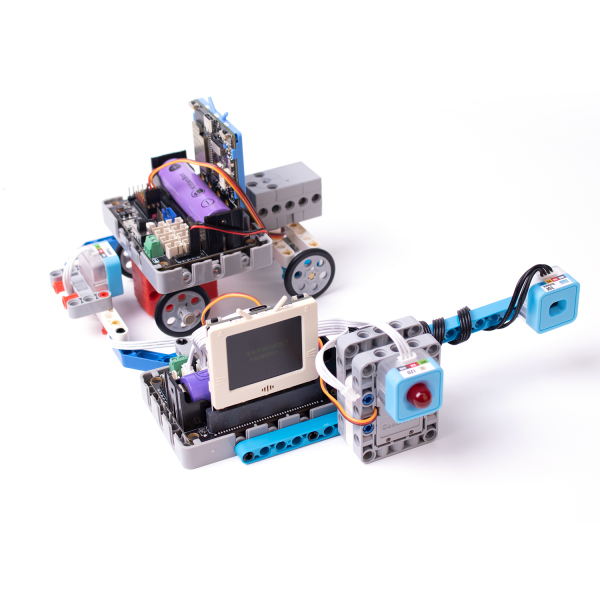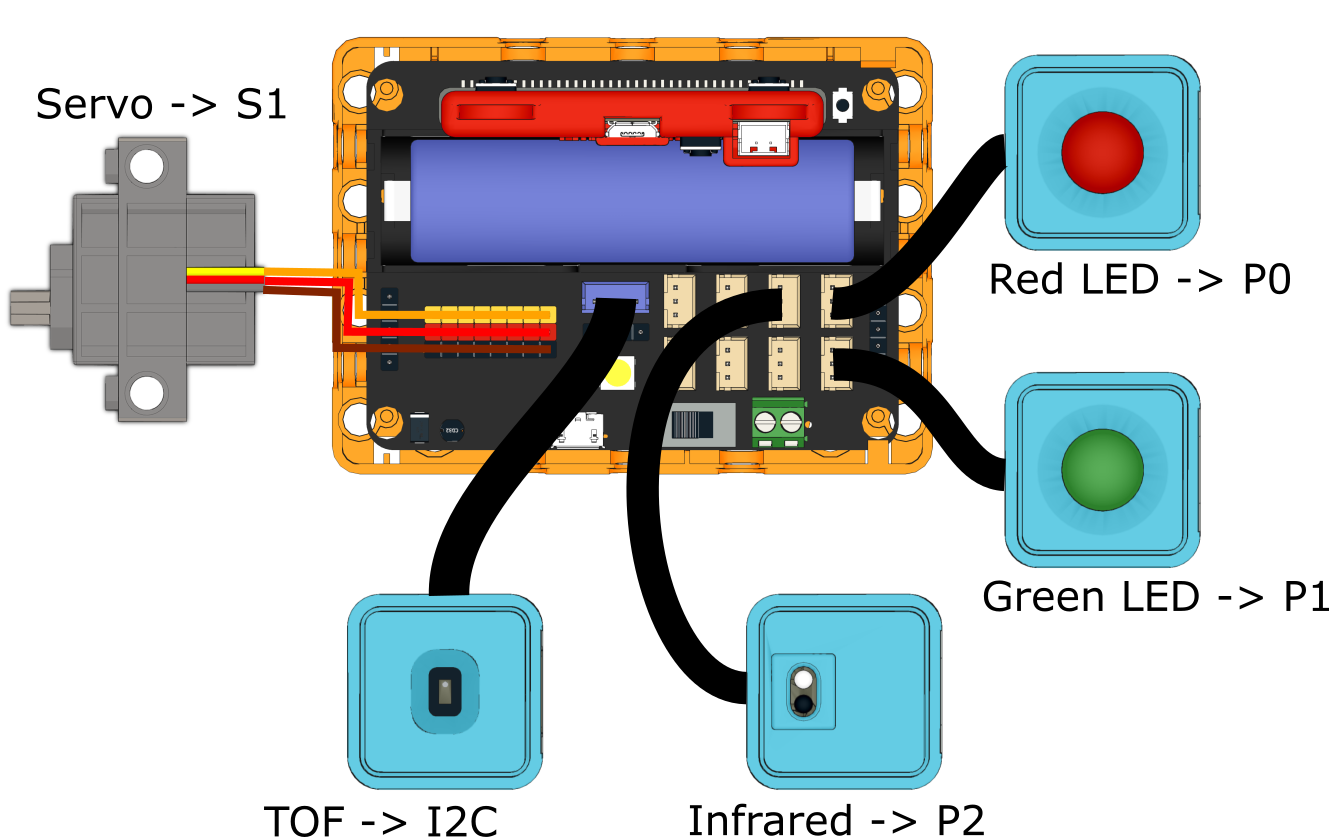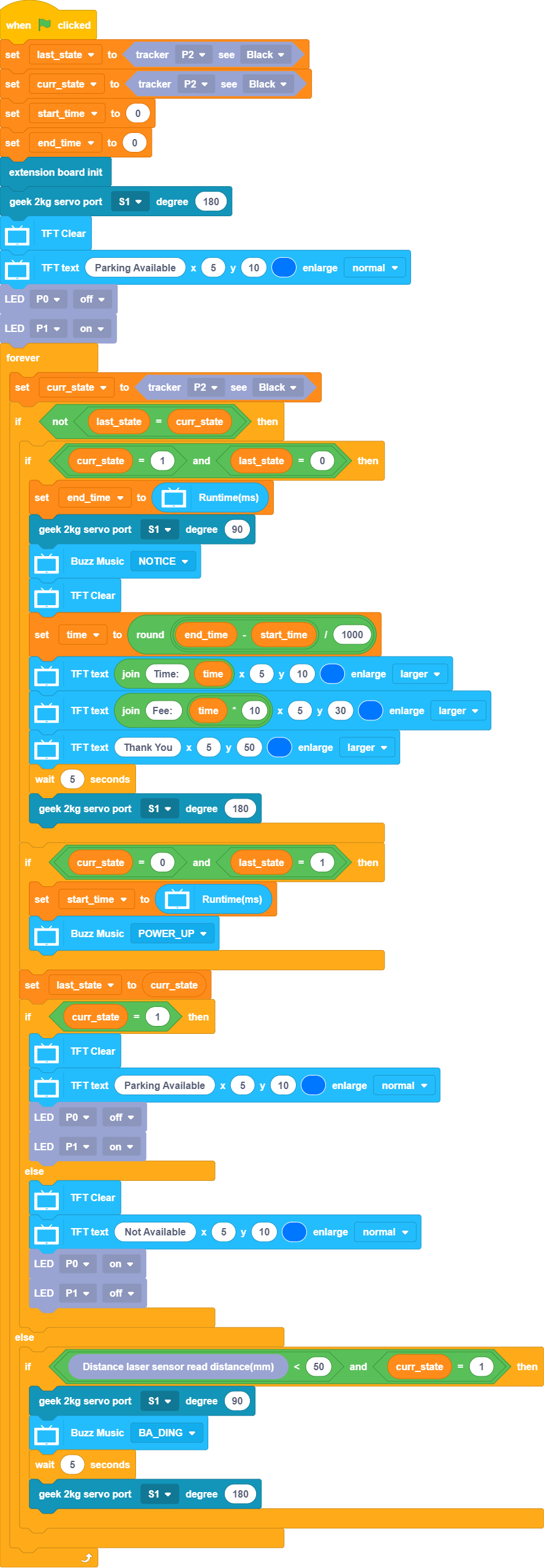# Smart Parking Lot

Simulate an autonomous parking lot, the gate opens and close automatically, number of available parking space is displayed on the FutureBoard.## Building Instructions

Building Instructions(Right Click->Save As)## Sample Program

### KittenBlock Sample Program### Python Sample Program

```#/bin/python

from time import sleep
from future import *
from sugar import *
import robotbit
import time

x = 0
last_state = 0
curr_state = 0
start_time = 0
end_time = 0
time = 0

last_state = Tracker("P2").value() == 1

curr_state = Tracker("P2").value() == 1

start_time = 0

end_time = 0

robot = robotbit.RobotBit()

robot.geekServo2kg(1, 180)

screen.clear()

screen.text(str("Parking Available"),5,10,1,(0, 119, 255))

LED("P0").state('OFF')

LED("P1").state('ON')

while True:
curr_state = Tracker("P2").value() == 1
if not last_state == curr_state:
if curr_state == 1 and last_state == 0:
end_time = time.ticks_ms()
robot.geekServo2kg(1, 90)
buzzer.melody(NOTICE)
screen.clear()
time = (round((end_time - start_time) / 1000))
screen.text(str(str("Time: ")+str(time)),5,10,2,(0, 119, 255))
screen.text(str(str("Fee: ")+str(time * 10)),5,30,2,(0, 119, 255))
screen.text(str("Thank You"),5,50,2,(0, 119, 255))
sleep(5)
robot.geekServo2kg(1, 180)
if curr_state == 0 and last_state == 1:
start_time = time.ticks_ms()
buzzer.melody(POWER_UP)
last_state = curr_state
if curr_state == 1:
screen.clear()
screen.text(str("Parking Available"),5,10,1,(0, 119, 255))
LED("P0").state('OFF')
LED("P1").state('ON')
else:
screen.clear()
screen.text(str("Not Available"),5,10,1,(0, 119, 255))
LED("P0").state('ON')
LED("P1").state('OFF')
else:
if TOFDistance().value() < 50 and curr_state == 1:
robot.geekServo2kg(1, 90)
buzzer.melody(BA_DING)
sleep(5)
robot.geekServo2kg(1, 180)
```